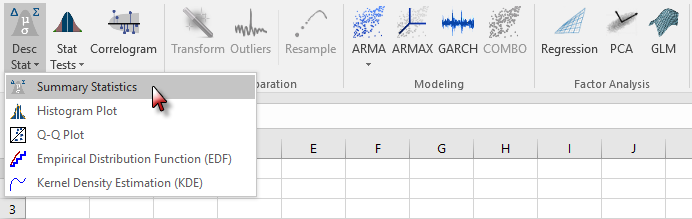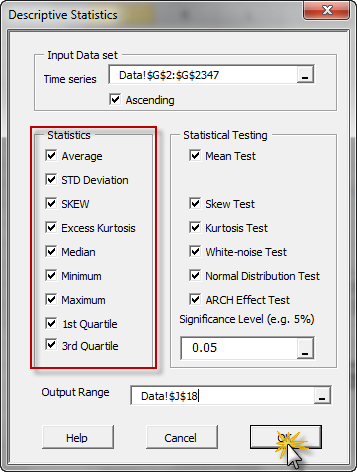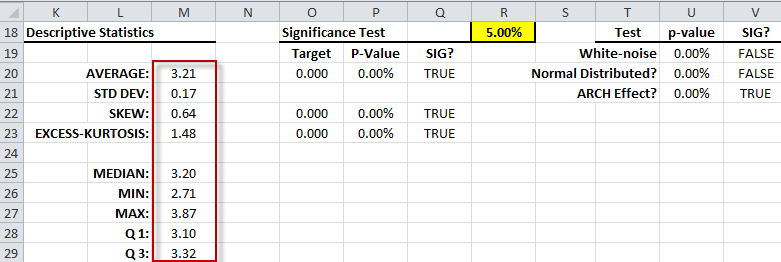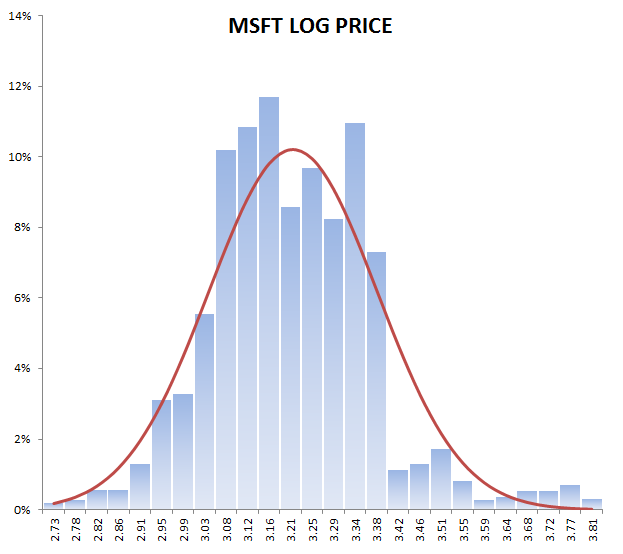# Module 2 - Descriptive Statistics

In module 1, we examined the MSFT daily closing prices time series to explore common issues associated with real-time series data: stationarity, homogeneity, outliers, missing values, and concentration of values. We concluded the module with a transformation of the input data using logarithmic transformation.

In this module, we will conduct a few computations to summarize the sample statistical distribution in an attempt to understand the unknown population distribution.

NumXL comes with scores of functions to compute various summary statistics functions, including robust functions like Quantile, IQR, etc. Furthermore, NumXL includes a wide range of statistical tests to verify the significance of the computed summary statistics.

## Illustration

For our purposes here, we’ll use the NumXL toolbar and summary statistics wizards. Using the NumXL tab, click DESC STAT.Next, the Descriptive Statistics dialog box will pop up. Fill in the fields with your (log-transformed) data location, series time order, options, and location for the results to appear on your worksheet.The Descriptive Statistics dialog box will print out the selected statistics and tests (along with the formulas) into your worksheet.In sum, one may conclude that the underlying distribution has the following properties:

• Mean is significantly different from zero
• Density (mass) distribution is significantly positively skewed
• Density distribution has fat-tails
• Half of the observation values fall between 3.09 and 3.32

Although the median is smaller than average, the distribution is positively skewed, leading us to believe that the distribution has the right fat-tails.

The quartiles (Q1, Q3) inscribe 50% of the values in the sample. The inter-quartile range (IQR) can be used to characterize the data when there may be extremities that skew the data; the interquartile range is a relatively robust statistic (also sometimes called "resistant") compared to the range and standard deviation.

We’ll revisit the statistical tests for white-noise, normality, and arch effect in module 5, but for now, we can ignore the right-most table.

Recall the MSFT log-price histogram in module 1 (see below):